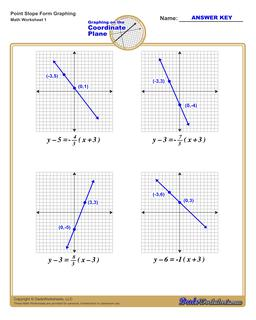One Dad. Four daughters. 9,393 worksheets... and counting!# Graph Point Slope Form

PropertyValue
DescriptionGraph Point Slope Form: Each graphing linear equations worksheet on this page has four coordinate planes and equations in point-slope form, and includes an answer key showing the correct graph.
Resource TypeWorksheet